# Filters and convolution

There are a set of custom made filters that can be used to apply filter effects on the image. These are `sharpen`, `emboss` and `blur`. These filters are applied last in the processing chain, after the image has been resized to its final dimensions.

## #Basics

The basics is to apply image processing based on a matrix, also called image convolution.

Sharpening an image enhances its edges and textures and makes it more focused and sharp.

Blurring an image makes it more unfocused, it smooths out the sharp edges.

Embossing makes the image look a little like 3D by adding highlights or shadows to the image.

In general, it seems like a good idea to add a sharpening effect when resizing images to smaller dimensions. Sharpening may remove the blurry edges that is a artifact from scaling down an image and result in an overall better looking image.

Examples on filtering
Original. `?w=300&save-as=jpg`Sharpen. `?w=300&save-as=jpg&sharpen`Emboss. `?w=300&save-as=jpg&emboss`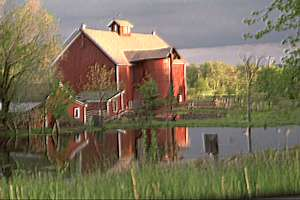Blur. `?w=300&save-as=jpg&blur`These are easy to use filters which quickly can add effects to the image. You can combine all three filters and they are then executed in this order, (1) blur, (2) emboss, (3) sharpen. These are the lasts effects that are applied to the image, just before saving it to disk.

## Custom filter with convolution

The filters above are implemented as convolve-expressions. These are a matrix of 3x3 together with a divisor and an offset. Its just like the PHP-function imageconvolution().

There are more convolution expressions supported. Lets see some examples on using convolution, based on this image.

Example of convolution
Lighten.
`?w=300&convolve=lighten`Darken.
`?w=300&convolve=darken`Sharpen.
`?w=300&convolve=sharpen`Sharpen-alt.
`?w=300&convolve=sharpen-alt`Blur.
`?w=300&convolve=blur`Gaussian blur.
`?w=300&convolve=gblur`Mean.
`?w=300&convolve=mean`Motion.
`?w=300&convolve=motion`Emboss.
`?w=300&convolve=emboss`Emboss alt.
`?w=300&convolve=emboss-alt`Edge.
`?w=300&convolve=edge`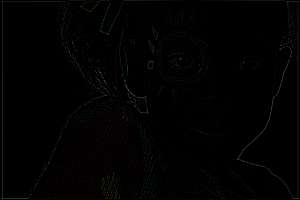Edge alt.
`?w=300&convolve=edge-alt`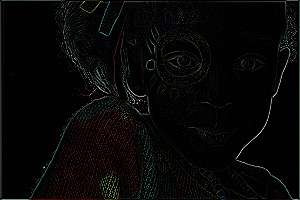Draw.
`?w=300&convolve=draw`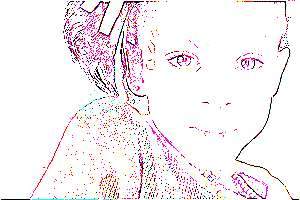Combine several filters.
`&convolve=draw:edge-alt:emboss-alt:motion`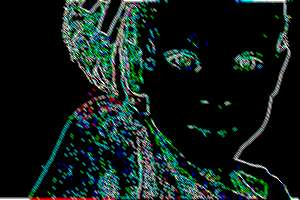If you have some special filter that you use a lot, then create a constant for it in `img_config.php`. One place to change it, for all images on your website.

## #Configuration of convolution expressions

CImage contains a set of convolution expressions. Here is the list.

```/**
* Custom convolution expressions, matrix 3x3, divisor and offset.
*/
private \$convolves = array(
'lighten'       => '0,0,0, 0,12,0, 0,0,0, 9, 0',
'darken'        => '0,0,0, 0,6,0, 0,0,0, 9, 0',
'sharpen'       => '-1,-1,-1, -1,16,-1, -1,-1,-1, 8, 0',
'sharpen-alt'   => '0,-1,0, -1,5,-1, 0,-1,0, 1, 0',
'emboss'        => '1,1,-1, 1,3,-1, 1,-1,-1, 3, 0',
'emboss-alt'    => '-2,-1,0, -1,1,1, 0,1,2, 1, 0',
'blur'          => '1,1,1, 1,15,1, 1,1,1, 23, 0',
'gblur'         => '1,2,1, 2,4,2, 1,2,1, 16, 0',
'edge'          => '-1,-1,-1, -1,8,-1, -1,-1,-1, 9, 0',
'edge-alt'      => '0,1,0, 1,-4,1, 0,1,0, 1, 0',
'draw'          => '0,-1,0, -1,5,-1, 0,-1,0, 0, 0',
'mean'          => '1,1,1, 1,1,1, 1,1,1, 9, 0',
'motion'        => '1,0,0, 0,1,0, 0,0,1, 3, 0',
);
```

Each expression is an eleven float value string, separated by commas. The string is converted like this, into a matrix, divisor and offset.

```    // As defined
'sharpen'  => '-1,-1,-1, -1,16,-1, -1,-1,-1, 8, 0',

// Converted to ([] is short syntax for array())
\$matrix = [
[-1, -1, -1],
[-1, 16, -1],
[-1, -1, -1],
];

\$divisor = 8;
\$offset  = 0;

// Called by this
\$img = imageconvolution(\$img, \$matrix, \$divisor, \$offset)
```

So, above expressions are defined in CImage. You can define your own in `img_config.php`. The default config-file contains an example on how to do it. They are outcommented by default since they are only there as an example.

```/**
* Create custom convolution expressions, matrix 3x3, divisor and
* offset.
*/
'convolution_constant' => array(
//'sharpen'       => '-1,-1,-1, -1,16,-1, -1,-1,-1, 8, 0',
//'sharpen-alt'   => '0,-1,0, -1,5,-1, 0,-1,0, 1, 0',
),
```

The convolution expressions defined in `img_config.php` will add to, or overwrite, those defined in CImage. Any convolution constant can then be used, no matter where its defined.